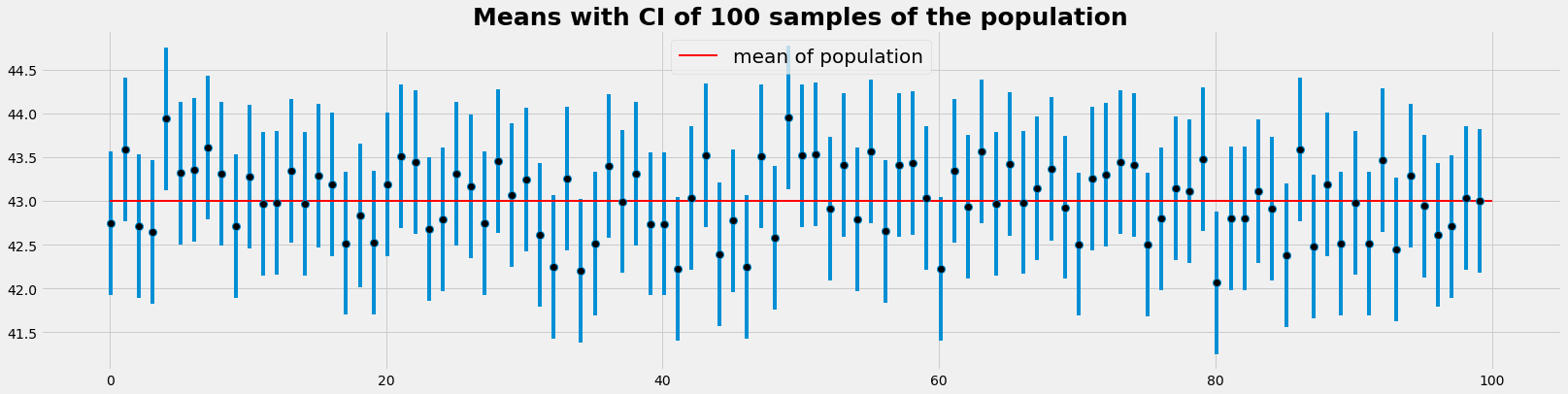.

.

.

.

.

# HealthyNumerics

HealthPoliticsEconomics | Quant Analytics | Numerics

# Articles

#### MachineLearning: Gradient descent from Nobel Laureates to chocolate consumption

Evaluating the appropriate parameters of a model is the core of every machine learning algorithm. In neural networks such a procedure has to be repeated over an over. Beceause of the non-linearities numerical approaches which approximate the solution iteratively are an important class of solution.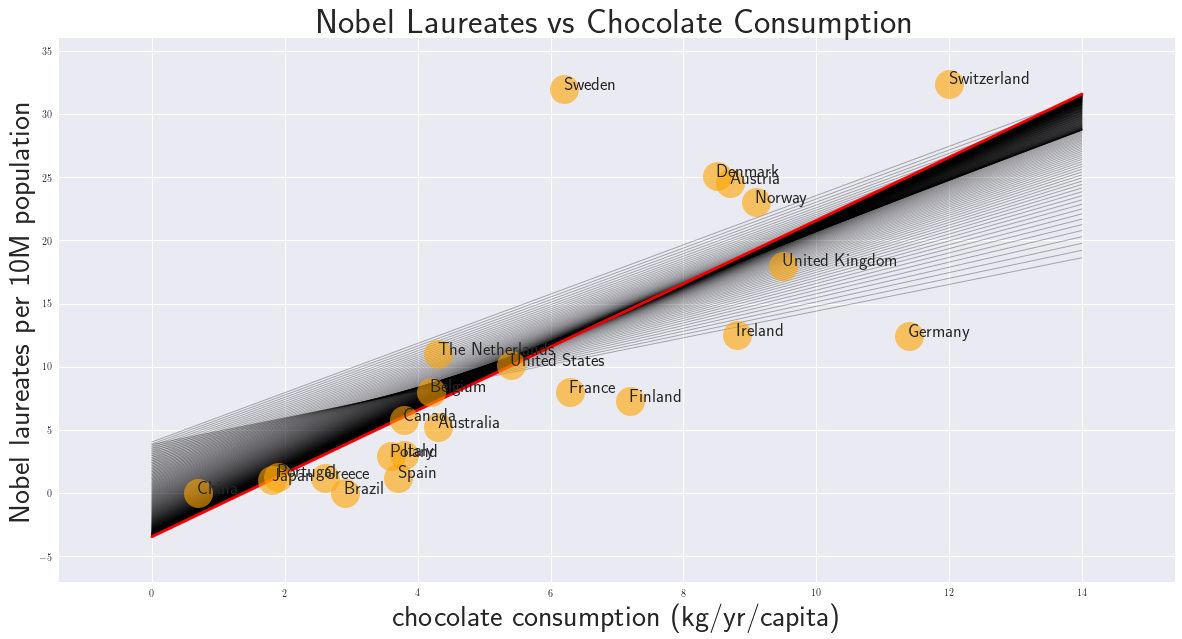#### MachineLearning: run through the basic steps

We go through the basic steps of machine learning using the most elementary models, linear and logistic regression namely. This gives a first outlook on the machine learning proces.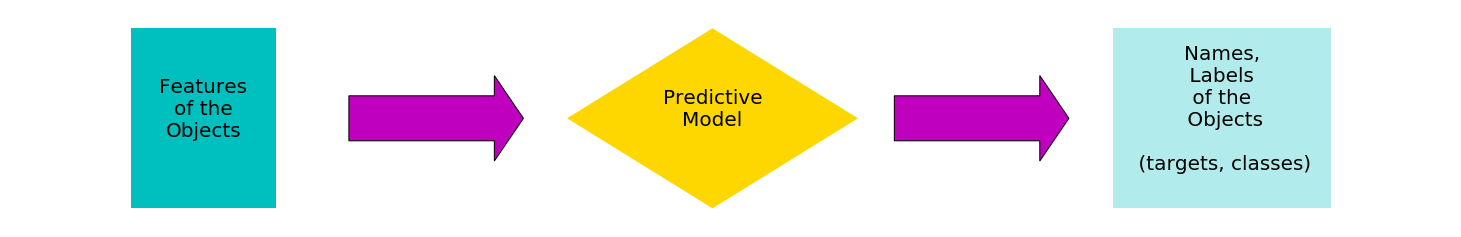#### The decorative side of mathematical functions

We start with a complex mathematical function and end up with some decorative graphics.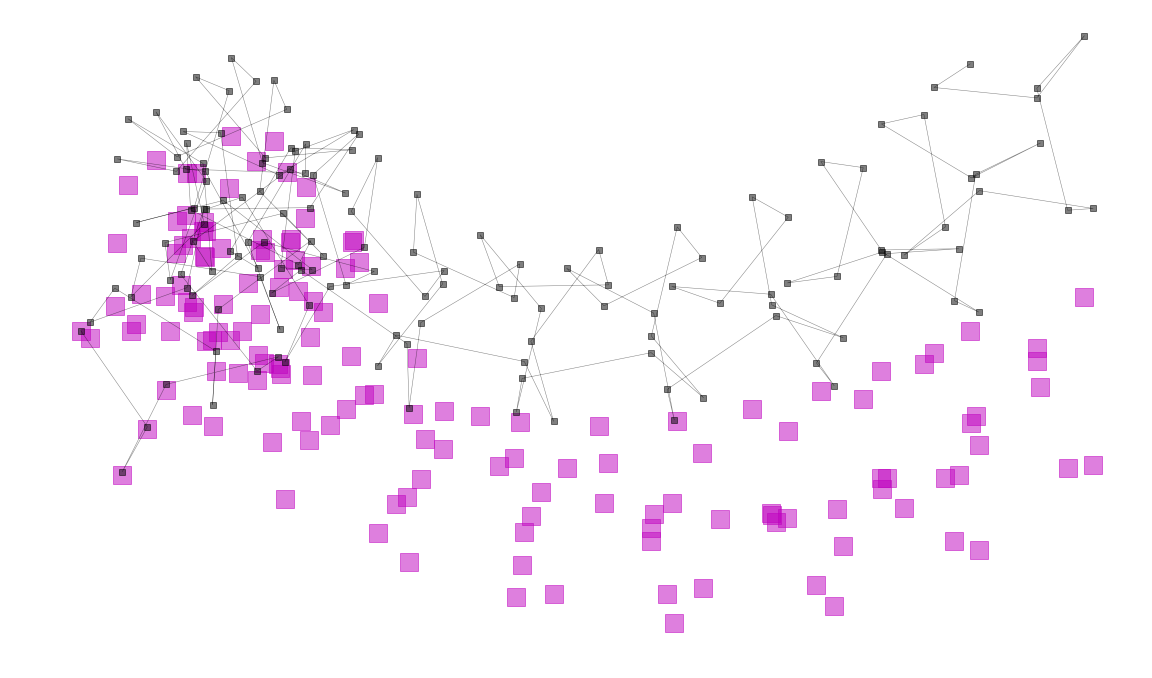#### An alpine landscape generator

Landscape generation using midpoint displacement in vectorized form#### Dynamics of heat flux with an inplicite time dependent FVM-model

We model the dynamics of solar heating and of the heat ground flux. We can reconstruct why the peak of heat is in the afternoon and not at noon (when the sun is in the zenith).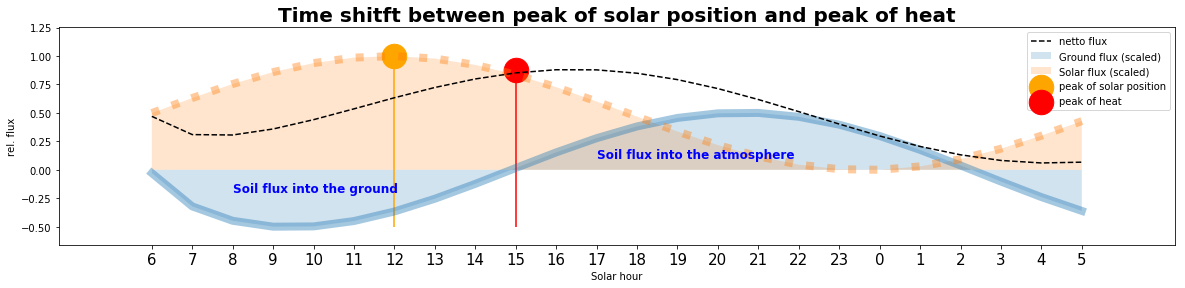#### An inplicite time dependent finite volume model (FVM) on an irregular grid for heat flow

We formulate the discretization of a heat flow model for an implicite finite volume method on an irregular grid and implement it for an 1-dimensional application. We give the detailled derivation from the partial differential equation to the FVM discretization and the implementation with vectorized Python code.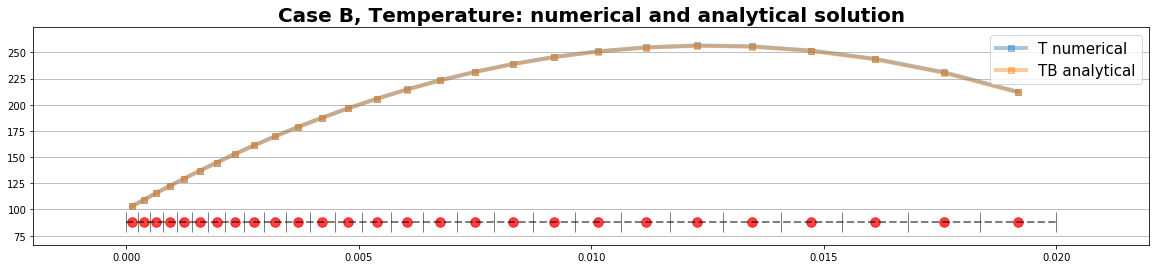#### 10 pictures Mondrian never has painted

A probalistic art engine for Mondrian style images#### An accelerated generator of pastel canvas

We try a beautiful art engine and use vectorized forms to accelerate the execution.#### The Fibonacci sequence in Mondrian style

A short code to plot the Fibonacci sequence as a curve.#### Convolution and Non-linear Regression

Two algorithms to determine the signal in noisy data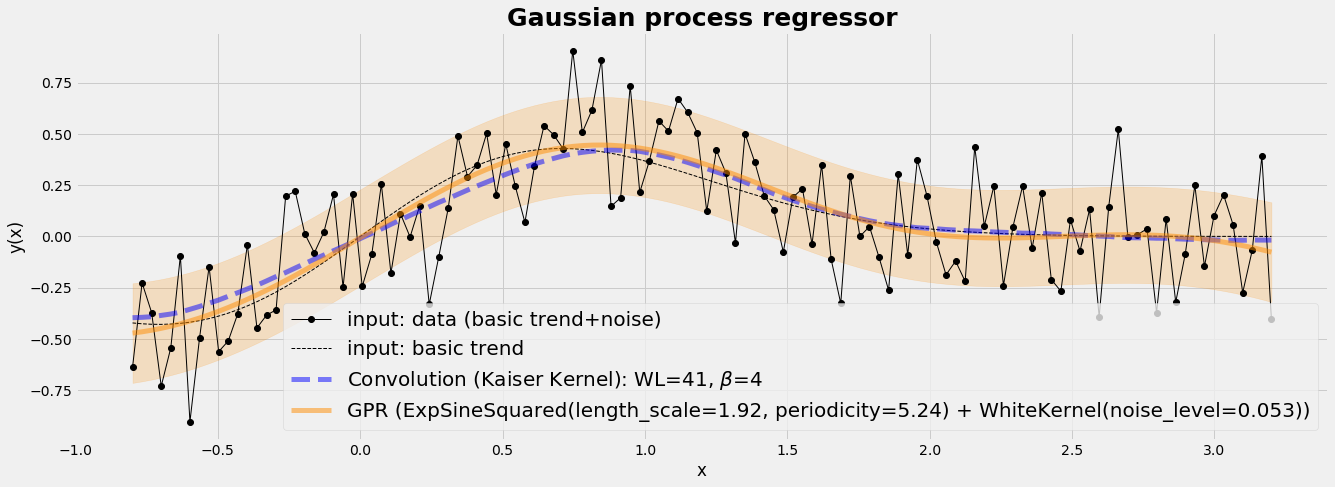#### Signal generation for distributions and heart beat (ECG wave)

We generate some basic signals and use convolution and windowing to re-construct ECG waves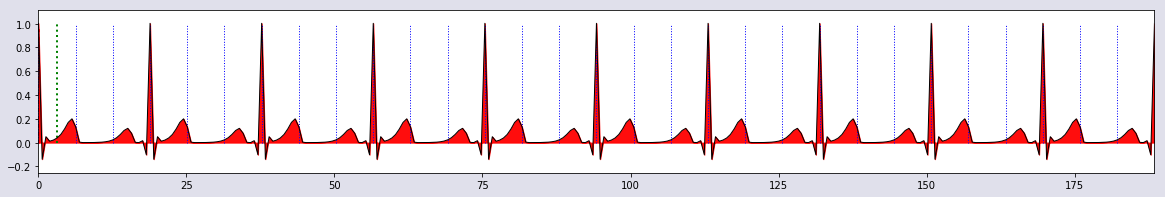#### Mapping of mean values and interpolation of distributions

We explore the building of mean values and interpolations when data have more than 1 dimension. We generate numerically a macro finite element so that arbitrary sized data can be anlalysed taking their inherent structure into account.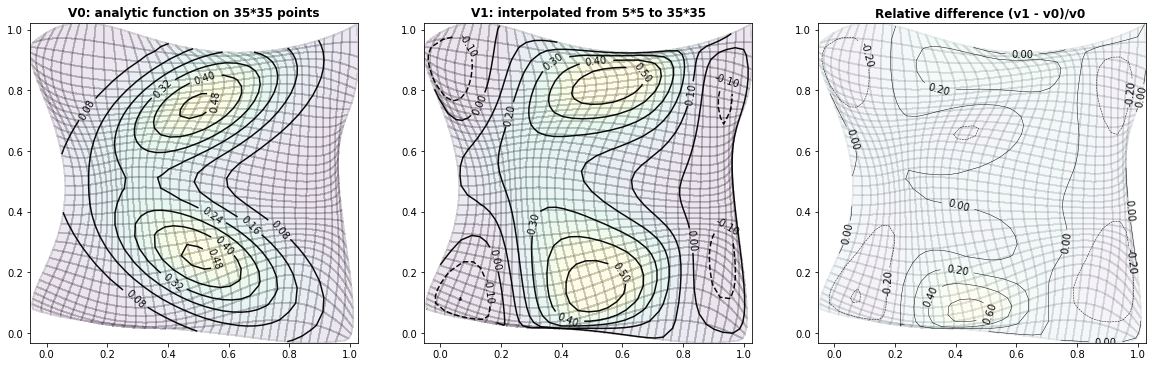#### NumericalStats: What the Metropolis Markov Chain Monte Carlo sampler is good for

We use the Metropolis-Hasting algorithm to sample a 2-dimensional empirical distribution.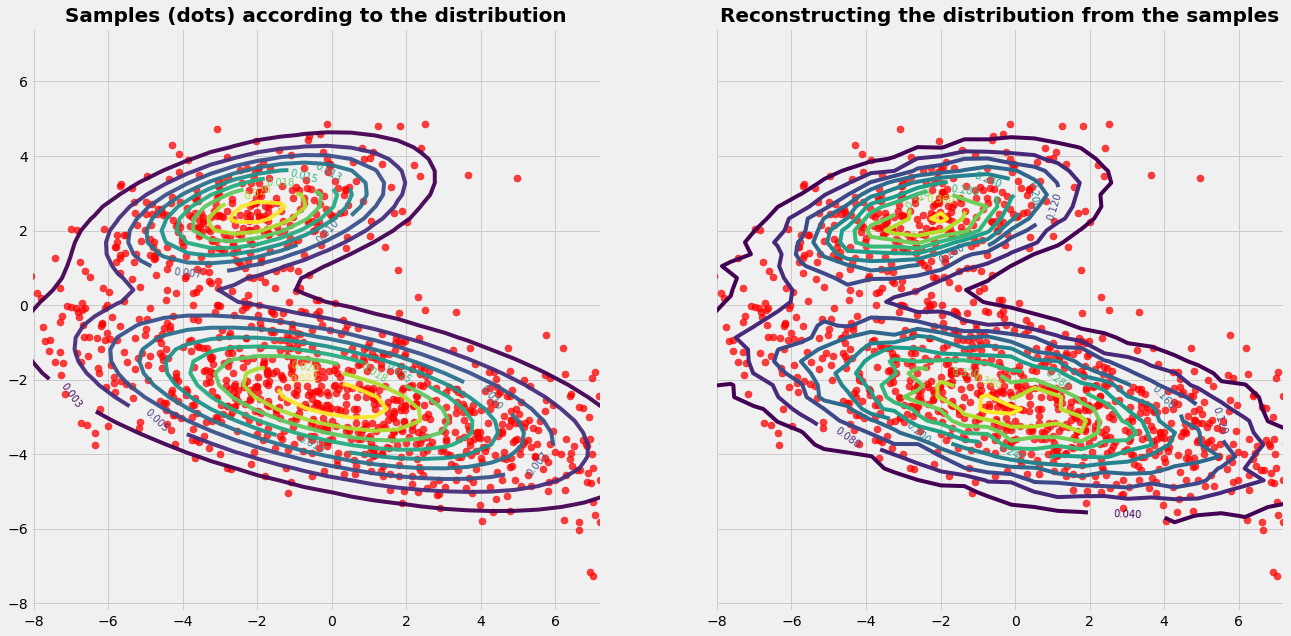#### How to climb the Matterhorn with a Markov Chain Monte Carlo walk

We let your robot climb the Matterhorn with a Markov Chain Monte Carlo walk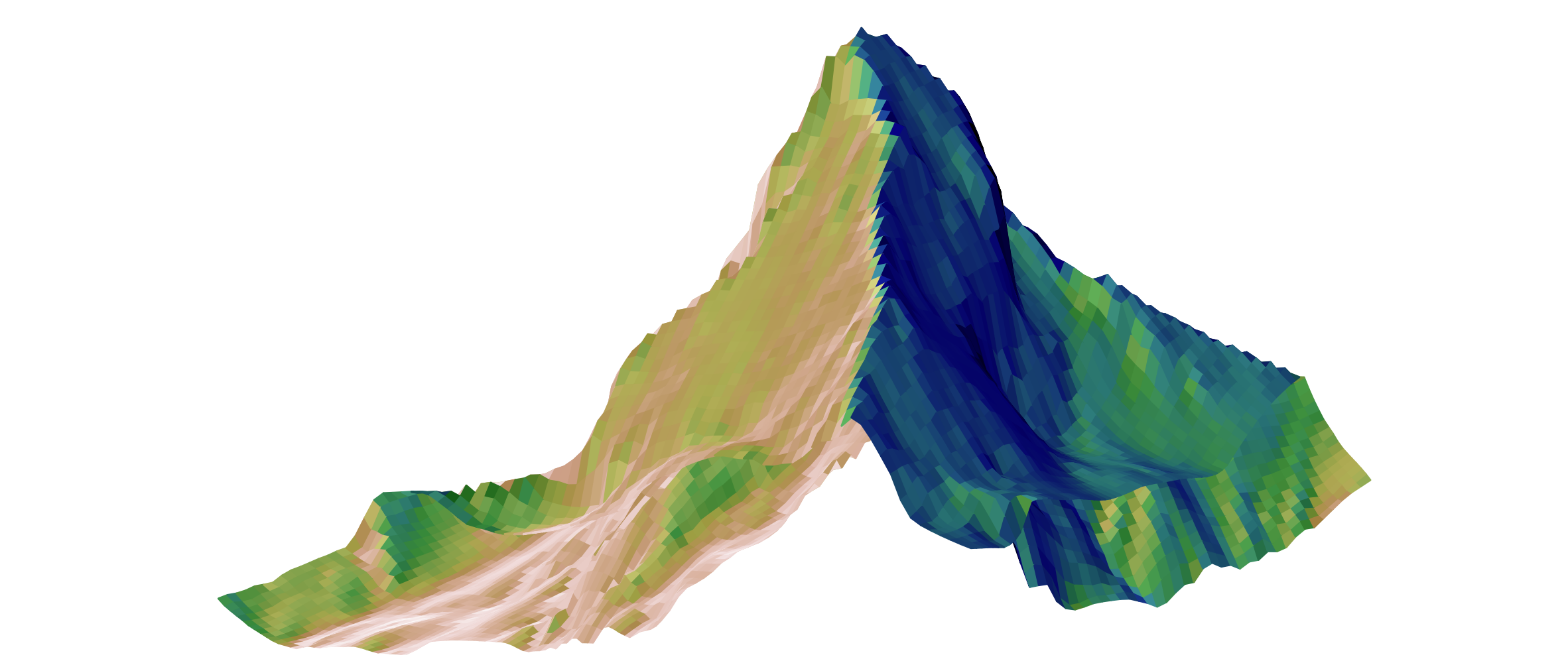#### Computational Fluid Dynamics: Markov Chain Monte Carlo in 2 dimensions

Stochastic modeling is a commonly used methodology in health economics and outcomes research (HEOR). We provide a visible insight in Markov Chain Monte Carlo modelling by a health related topic, the distribution of pollutants.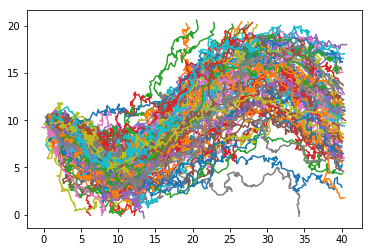#### MachineLearning: How to analyze longitudinal studies with time series clustering

With the increasing possibilities to gather longitudinal data, there is an interest in mining profiles in form of time series data. The key question is how to figure out and to group similarities and dissimilarities between the profiles.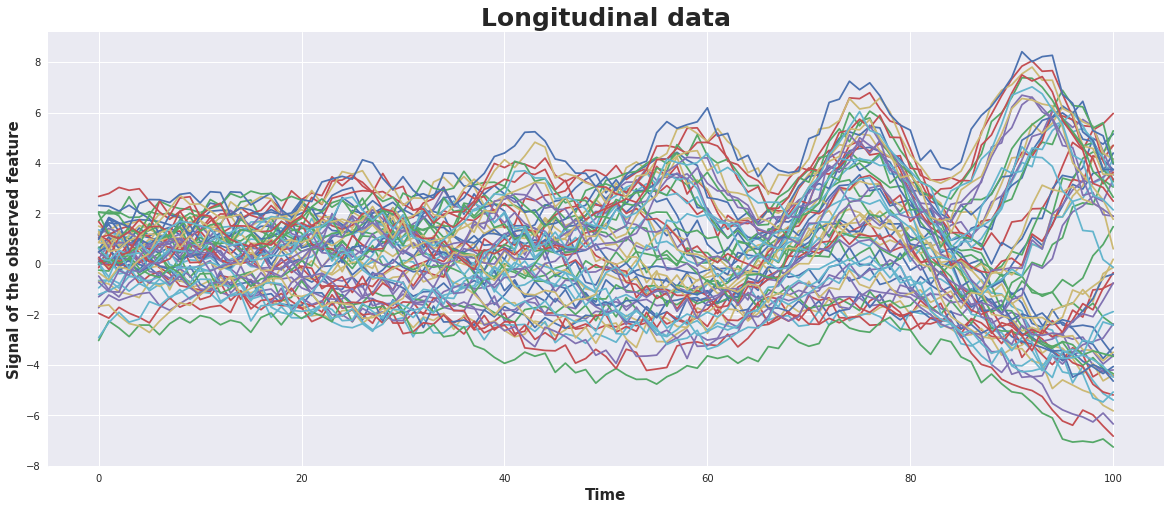#### NumericalStats: WHY and HOW to involve medical researchers into the statistical model building

The Monty Hall problem came out of a US TV-game in the 1970's and got wide publicity about problem solving statistically. It can be transfered easely to medical research how to seek a strategy that proofs to be the most suitable to reach ceratin aims in healthcare.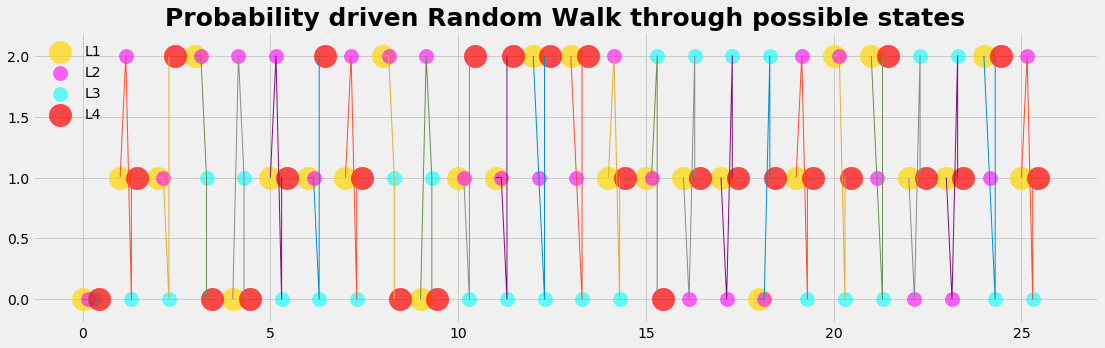#### NumericalStats: WHY to switch to distribution vectors

Using the coin flipping example, we give some arguments WHY the use of distribution vectors can be helpful as a preparation for Monte Carlo Markov Chain models and others and how this changes the role of medical researchers.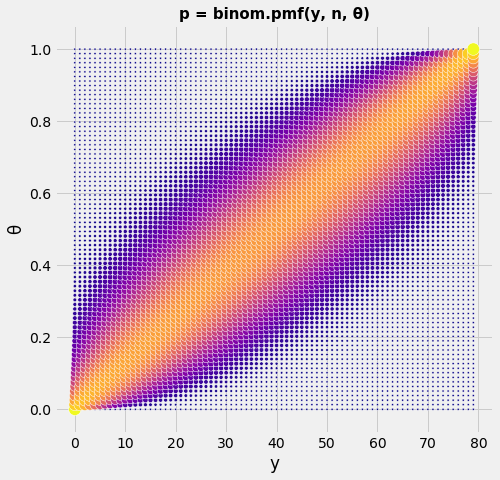#### NumericalStats: WHY to switch from a decision tree to a Markov model

We give some arguments, why a change from a decision tree to a Markov model could be motivated. We provide a code of 7 lines to run a Markov model.#### MetaAnalysis: Evaluating a diagnostic test with continuous output

The output of a dignostic test is often not binary but continuous. The transformation of the continuous output into a binary variable influences the outcome of the test.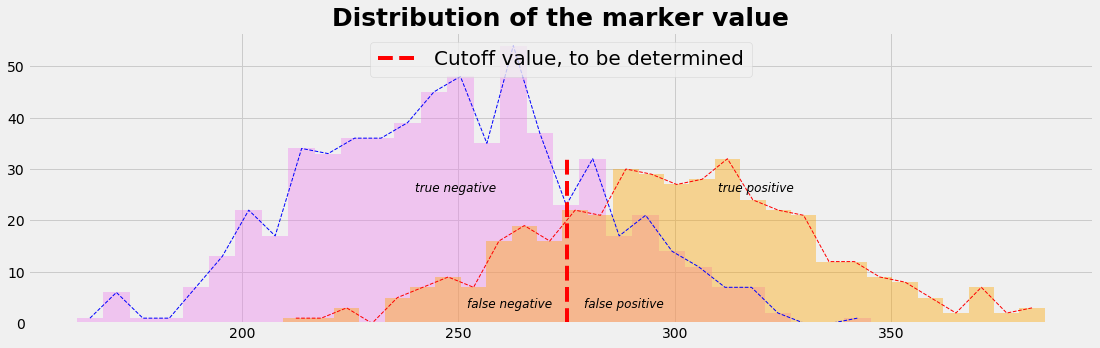#### MetaAnalysis: Screening with a binary diagnostic test

Given a diagnostic test with sensitivity 0.90 and specifity 0.95. Then, with a prevalence of 0.02, in medical practice 27% of the positive tests will be right and 73% of the positive tests will be wrong.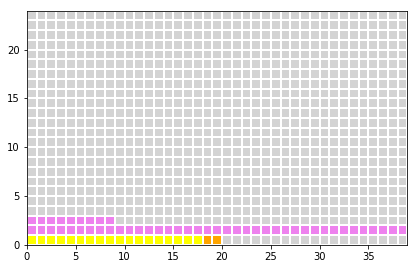#### "America first, Switzerland second" - geschafft !

Die OECD-Daten zeigen, dass das US-Gesundheitssystem nicht mit dem Rest der Welt mithalten kann. Es ist das teuerste System, gefolgt von der Schweiz.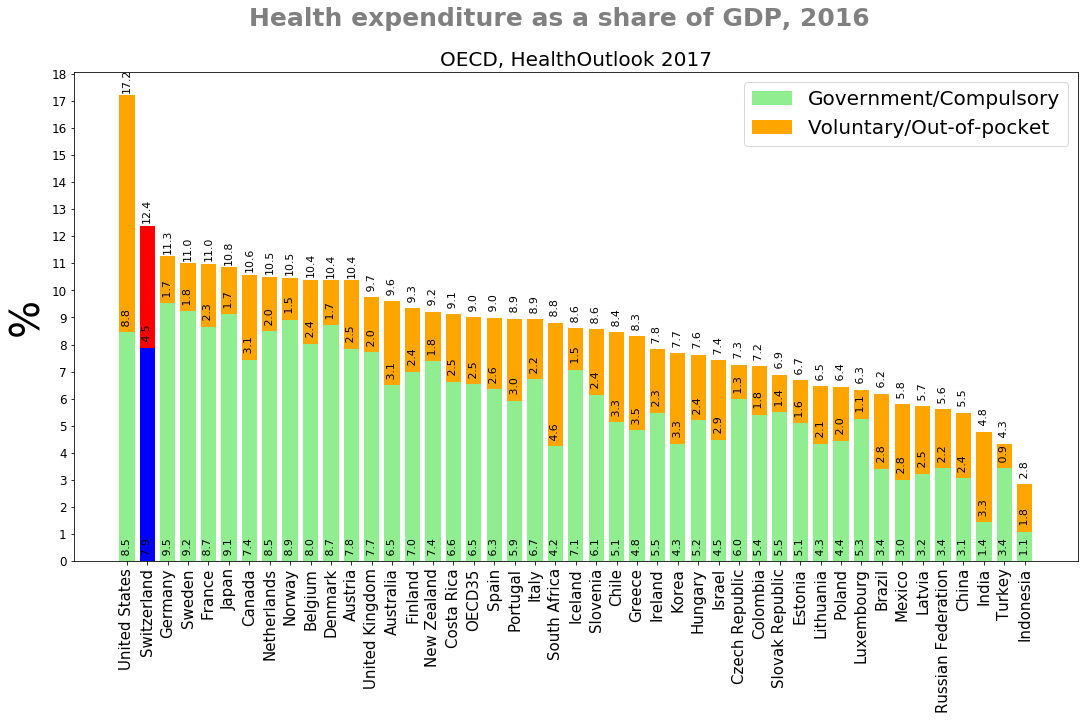#### VIDEO: Bevölkerungsdynamik Schweiz 1876 - 2014

Von der Bevölkerungspyramide zur Bevökerumgstanne - Dynamik von Geburten und Bevölkerungsentwicklung in der Schweiz von 1876 bis 2014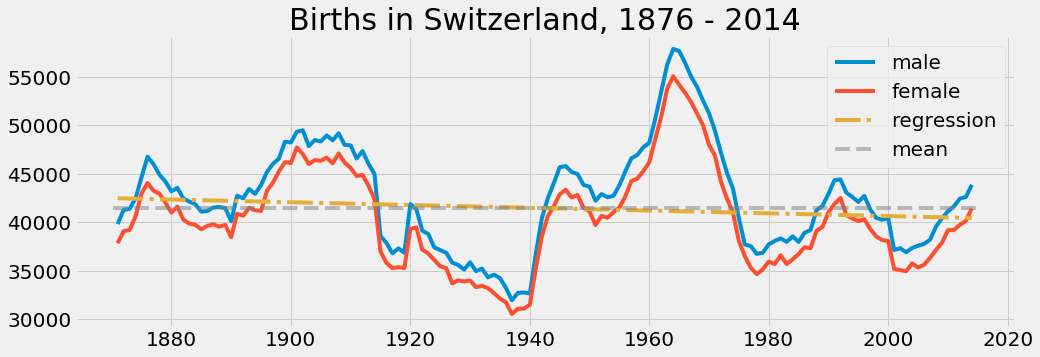#### NumericalStats: How to randomly sample your empirical arbitrary distribution

We provide a simple sampling engine which allows to generate random numbers that are distributed as an empirical and arbitrary distribution given as a data array.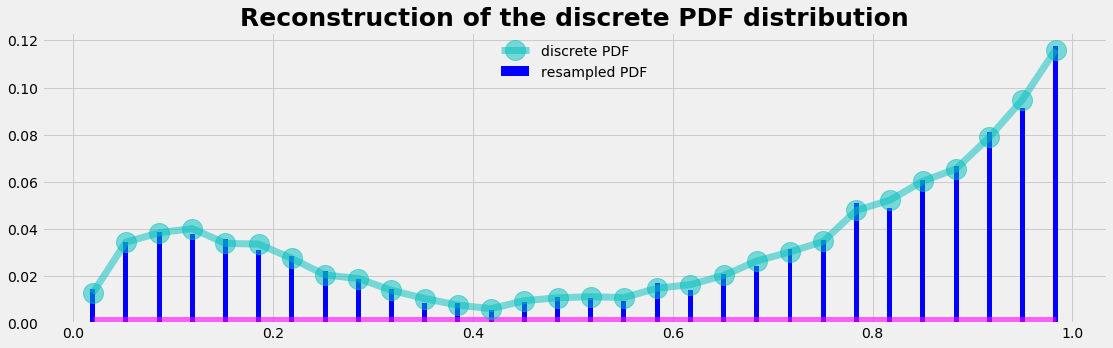#### BasicStats: The secret of the CI

We reproduce numerically the meaning of the confidential intervall